# 5.2 Solve systems of equations by substitution  (Page 4/5)

 Page 4 / 5

The perimeter of a rectangle is 40. The length is 4 more than the width. Find the length and width of the rectangle.

The length is 12 and the width is 8.

The perimeter of a rectangle is 58. The length is 5 more than three times the width. Find the length and width of the rectangle.

The length is 23 and the width is 6.

For [link] we need to remember that the sum of the measures of the angles of a triangle is 180 degrees and that a right triangle has one 90 degree angle.

The measure of one of the small angles of a right triangle is ten more than three times the measure of the other small angle. Find the measures of both angles.

## Solution

We will draw and label a figure.

 Step 1. Read the problem.Step 2. Identify what you are looking for. We are looking for the measures of the angles. Step 3. Name what we are looking for. Let $a=$ the measure of the 1 st angle $\phantom{\rule{1.5em}{0ex}}b=$ the measure of the 2 nd angle Step 4. Translate into a system of equations. The measure of one of the small angles of a right triangle is ten more than three times the measure of the other small angle.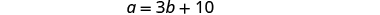The sum of the measures of the angles of a triangle is 180.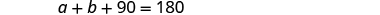The system is: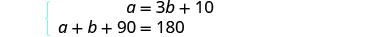Step 5. Solve the system of equations. We will use substitution since the first equation is solved for a .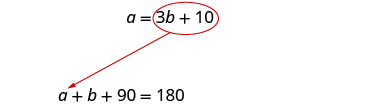Substitute 3 b + 10 for a in the second equation.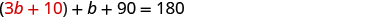Solve for b .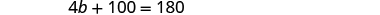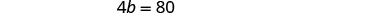Substitute b = 20 into the first equation and then solve for a .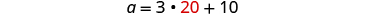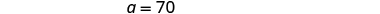Step 6. Check the answer in the problem. We will leave this to you! Step 7. Answer the question. The measures of the small angles are 20 and 70.

The measure of one of the small angles of a right triangle is 2 more than 3 times the measure of the other small angle. Find the measure of both angles.

The measure of the angles are 22 degrees and 68 degrees.

The measure of one of the small angles of a right triangle is 18 less than twice the measure of the other small angle. Find the measure of both angles.

The measure of the angles are 36 degrees and 54 degrees.

Heather has been offered two options for her salary as a trainer at the gym. Option A would pay her $25,000 plus$15 for each training session. Option B would pay her $10,000 +$40 for each training session. How many training sessions would make the salary options equal?

## Solution

 Step 1. Read the problem. Step 2. Identify what you are looking for. We are looking for the number of training sessions that would make the pay equal. Step 3. Name what we are looking for. Let $s=$ Heather’s salary. $\phantom{\rule{1.5em}{0ex}}n=$ the number of training sessions Step 4. Translate into a system of equations. Option A would pay her $25,000 plus$15 for each training session.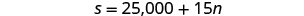Option B would pay her $10,000 +$40 for each training session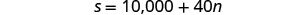The system is: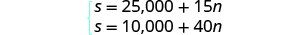Step 5. Solve the system of equations. We will use substitution.Substitute 25,000 + 15 n for s in the second equation.Solve for n .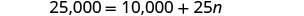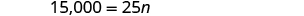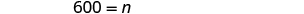Step 6. Check the answer. Are 600 training sessions a year reasonable? Are the two options equal when n = 600? Step 7. Answer the question. The salary options would be equal for 600 training sessions.

Geraldine has been offered positions by two insurance companies. The first company pays a salary of $12,000 plus a commission of$100 for each policy sold. The second pays a salary of $20,000 plus a commission of$50 for each policy sold. How many policies would need to be sold to make the total pay the same?

There would need to be 160 policies sold to make the total pay the same.

Aziza is solving this equation-2(1+x)=4x+10
No. 3^32 -1 has exactly two divisors greater than 75 and less than 85 what is their product?
x^2+7x-19=0 has Two solutions A and B give your answer to 3 decimal places
3. When Jenna spent 10 minutes on the elliptical trainer and then did circuit training for20 minutes, her fitness app says she burned 278 calories. When she spent 20 minutes onthe elliptical trainer and 30 minutes circuit training she burned 473 calories. How manycalories does she burn for each minute on the elliptical trainer? How many calories doesshe burn for each minute of circuit training?
.473
Angelita
?
Angelita
John left his house in Irvine at 8:35 am to drive to a meeting in Los Angeles, 45 miles away. He arrived at the meeting at 9:50. At 3:30 pm, he left the meeting and drove home. He arrived home at 5:18.
p-2/3=5/6 how do I solve it with explanation pls
P=3/2
Vanarith
1/2p2-2/3p=5p/6
James
Cindy
4.5
Ruth
is y=7/5 a solution of 5y+3=10y-4
yes
James
Cindy
Lucinda has a pocketful of dimes and quarters with a value of $6.20. The number of dimes is 18 more than 3 times the number of quarters. How many dimes and how many quarters does Lucinda have? Rhonda Reply Find an equation for the line that passes through the point P ( 0 , − 4 ) and has a slope 8/9 . Gabriel Reply is that a negative 4 or positive 4? Felix y = mx + b Felix if negative -4, then -4=8/9(0) + b Felix -4=b Felix if positive 4, then 4=b Felix then plug in y=8/9x - 4 or y=8/9x+4 Felix Macario is making 12 pounds of nut mixture with macadamia nuts and almonds. macadamia nuts cost$9 per pound and almonds cost $5.25 per pound. how many pounds of macadamia nuts and how many pounds of almonds should macario use for the mixture to cost$6.50 per pound to make?
Nga and Lauren bought a chest at a flea market for $50. They re-finished it and then added a 350 % mark - up Makaila Reply$1750
Cindy
the sum of two Numbers is 19 and their difference is 15
2, 17
Jose
interesting
saw
4,2
Cindy
Felecia left her home to visit her daughter, driving 45mph. Her husband waited for the dog sitter to arrive and left home 20 minutes, or 13 hour later. He drove 55mph to catch up to Felecia. How long before he reaches her?
hola saben como aser un valor de la expresión
NAILEA
integer greater than 2 and less than 12
2 < x < 12
Felix
I'm guessing you are doing inequalities...
Felix
Actually, translating words into algebraic expressions / equations...
Felix
hi
Darianna
hello
Mister
Eric here
Eric
6
Cindy

#### Get Jobilize Job Search Mobile App in your pocket Now!By OpenStaxBy Rebecca ButterfieldBy OpenStaxBy OpenStaxBy OpenStaxBy Janet ForresterBy Hannah ShethBy Hope PercleBy OpenStaxBy JavaChamp Team